# ISEE Middle Level Math : Median

## Example Questions

### Example Question #21 : Median

Find the median in this set of numbers: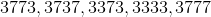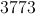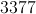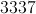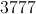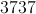Explanation:

First, order the numbers from least to greatest: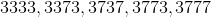Then, identify the middle number: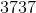### Example Question #22 : Median

What is the median of the following numbers?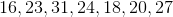22.7

23

15

20

23

Explanation:

Reorder the values in numberical order: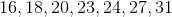The number in the center (23) is the median. If there are 2 center numbers, take the average of them to find the median.

### Example Question #23 : How To Find Median

Find the median in this set of numbers: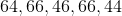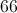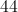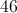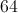Explanation:

First place the numbers in order from least to greatest: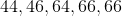Identify the middle number:### Example Question #24 : How To Find Median

Find the median in this set of numbers: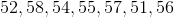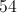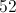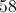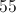Explanation:

First, order the numbers from least to greatest: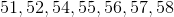Identify the middle number:### Example Question #25 : How To Find Median

Find the median in this set of numbers: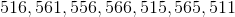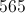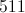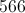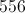Explanation:

First, put the numbers in order from least to greatest: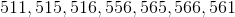Then, identify the middle number: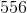### Example Question #26 : How To Find Median

Find the median in this set of numbers: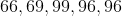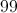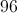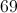Explanation:

First, order the numbers from least to greatest: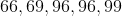Then, identify the middle number:### Example Question #27 : How To Find Median

Find the median in this set of numbers: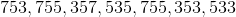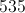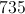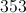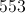Explanation:

First, order the numbers from least to greatest: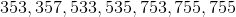Then, identify the middle number: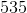### Example Question #1 : How To Find Median

Consider the data set: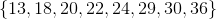What is the difference between the mean of this set and the median of this set?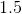Explanation:

To get the mean, add the numbers and divide by 8: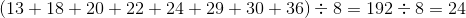To get the median, find the mean of the fourth- and fifth-highest elements (the ones in the middle):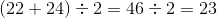The difference is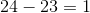### Example Question #29 : How To Find Median

Find the median in this set of numbers: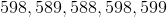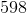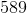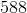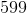Explanation:

First, order the numbers from least to greatest: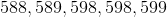Then, identify the middle number:### Example Question #30 : How To Find Median

Find the median in this set of numbers: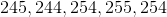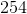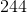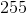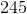Explanation:

First, order the numbers from least to greatest: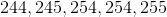Then, identify the middle number: